# Power Laws, Pareto Distribution and Zipf's Law

Summarizing: [newman-2006-archiv:cond-mat]

Many measured quantities concentrate around a mean value.

• heights of human beings: adult human beings average about 180cm. Tallest and shortest adult men as having had heights 272cm and 57cm (ratio 4.8).
• speeds in miles per hour of cars on British highways are strongly peaked around 75mph.

# Centered Distributions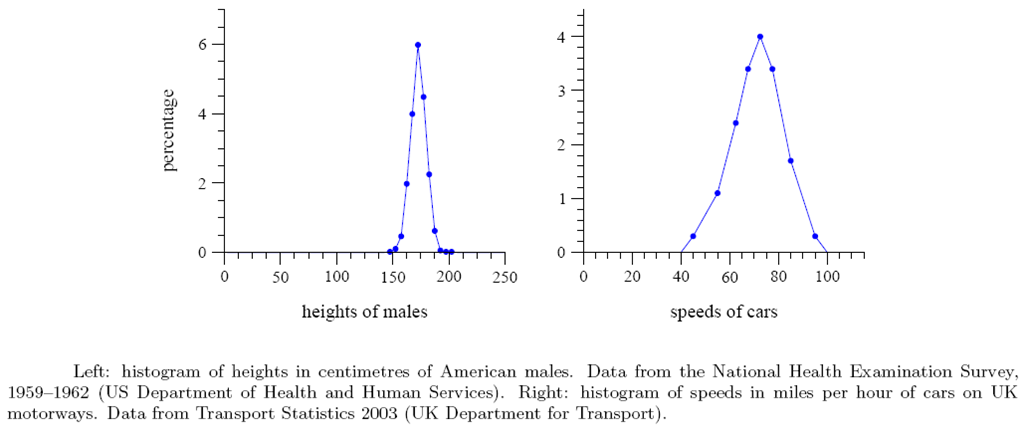# Other Distributions

BUT not all measured concentrate nicely around a mean.

Some vary over an enormous range (sometimes many orders of magnitude). E.g., 2700 US city populations.

• largest population of any city in the US is 8.00 million for New York City (2000)
• America’s smallest town is Duffield, Virginia, with a population of 52
• the ratio of largest to smallest population is at least 150 000.

America’s total population of 300 million people could be allocated to 40 cities the size of New York. But America’s 2700 cities cannot have a mean population of more than 110,000.

# City Populations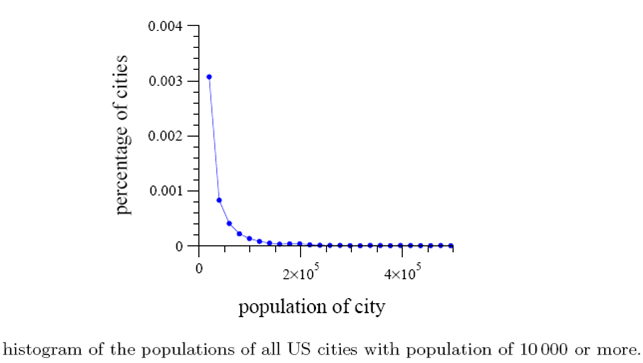Source: Newman (2006)

# City Populations

A histogram of city sizes plotted with logarithmic horizontal and vertical axes follows quite closely a straight line.

# City Populations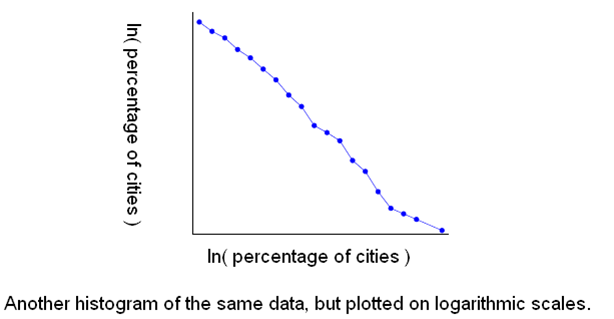Source: Newman (2006)

# Power Law Distribution

$\begin{array}{c}lny=-alnx+c\end{array}$
$\begin{array}{c}f\left(x\right)=c{x}^{-a}\end{array}$

Power law implies that small $x$ are very common and large $x$ are quite rare.

(See Mathematica notebook.)

# Pareto Distribution

• power law probability distribution
• named after Vilfredo Pareto (1848-1923, Italian economist)
• also known as Bradford distribution

Note: these notes draw on http://en.wikipedia.org/wiki/Pareto_distribution, http://www.math.uah.edu/stat/special/Pareto.pdf,

# Define Pareto Distribution

For $x\ge {x}_{m}$ we have

$\begin{array}{c}Pr\left(X>x\right)={\left(\frac{{x}_{m}}{x}\right)}^{\alpha }\end{array}$
• parameterized by ${x}_{m}$ and $\alpha$, where ${x}_{m}>0$ is the cutoff (minimum possible realization), and $\alpha >0$ is called the Pareto index or shape parameter.
• we can define the probability function below the cutuff: $Pr\left(X>x\right)=1$ for $x<{x}_{m}$
• the basic Pareto distribution sets ${x}_{m}=1$
• interpret $Pr\left(X>x\right)$ as the fraction of the population with wealth greater than $x$.

# CDF

For $x\ge {x}_{m}$

$\begin{array}{c}{F}_{X}\left(x\right)=1-{\left(\frac{{x}_{m}}{x}\right)}^{\alpha }\end{array}$

# Density function

Differentiating w.r.t. $x$, the probability density function is

$\begin{array}{c}{f}_{X}\left(x\right)=\alpha \frac{{x}_{m}^{\alpha }}{{x}^{\alpha +1}}\end{array}$

# Moments (Mean and Variance)

If $\alpha \le 1$, the expected value is infinite. If $\alpha >1$ then

$\begin{array}{c}E\left(X\right)=\frac{\alpha {x}_{m}}{\alpha -1}\end{array}$

If $\alpha \le 2$, the variance does not exist. If $\alpha >2$ then

$\begin{array}{c}var\left(X\right)={\left(\frac{{x}_{m}}{\alpha -1}\right)}^{2}\frac{\alpha }{\alpha -2}.\end{array}$

The raw moment moments are

$\begin{array}{c}{\mu }_{n}\text{'}=\frac{\alpha {x}_{m}^{n}}{\alpha -n}\end{array}$

A finite $n$-th moment exists only for $n<\alpha$.

# Conditional Distribution

Here is a cool property of the Pareto variate. The conditional probability

$\begin{array}{c}Pr\left(X>x|X>{x}_{1}\ge {x}_{m}\end{array}$

is also a Pareto distribution. It has the same Pareto index $\alpha$ but has cutoff ${x}_{1}$.

# Applications

(selected from Wikipedia)

• sizes of human settlements (few cities, many hamlets/villages)
• file size distribution of internet traffic
• values of oil reserves in oil fields (a few large fields, many small fields)
• length distribution in jobs assigned supercomputers (a few large ones, many small ones)
• areas burnt in forest fires

# Application to Wealth and Income

Wealth and income distribution
• pdf shows that the "probability" or fraction of the population that owns a small amount of wealth per person is rather high, and then decreases steadily as wealth increases.
• Pareto used this to describe the distribution of wealth and of income among individuals
• most wealth of any society is owned by a small percentage of the people in that society
• Pareto principle: "80-20 rule" (20% of the population controls 80% of the wealth)

# Pareto principle

The 80-20 law holds when the Pareto index is $\alpha =lo{g}_{4}5$.

80-20 law
• 20% of all people receive 80% of all income
• make a group of the top 20% → 20% of the group receive 80% of its income
• recursively

(Excludes $0<\alpha \le 1$, which implies infinite expected value and thus cannot model income distribution.)

# Relation to Zipf's law

Zipf's law

• the zeta distribution
• discrete analog of Pareto distribution
• the $n$-th largest value of X is approximately $C{n}^{-k}$

# Relation to the exponential distribution

The Pareto distribution is related to the exponential distribution as follows. If $X$ is Pareto-distributed with minimum ${x}_{m}$ and index $\alpha$, then

$\begin{array}{c}Y=ln\left(\frac{X}{{x}_{m}}\right)\end{array}$

is exponentially distributed with intensity $\alpha$. Equivalently, if $Y$ is exponentially distributed with intensity $\alpha$, then

$\begin{array}{c}{x}_{m}{e}^{Y}\end{array}$

is Pareto-distributed with minimum ${x}_{m}$ and index $\alpha$.

# Lorenz Curve (background)

Let $f$ be any pdf with finite mean $\mu =\underset{{x}_{m}}{\overset{\infty }{\int }}xf\left(x\right)dx$. Then the associated quantile function (Lorenz curve) $L\left(F\right)$ can be written as

$\begin{array}{c}L\left(F\right)=\frac{\underset{{x}_{m}}{\overset{x\left(F\right)}{\int }}xf\left(x\right)dx}{\mu }\end{array}$

Here $x\left(F\right)$ is the inverse of the CDF. Recall $F\left(x\right)$ is the fraction of the population with wealth no bigger than \$x\$, so $x\left(F\right)$ gives the maximum wealth found in that subpopulation. So the numerator is this subpopulations average wealth. The denominator is the average wealth of the population.

Correspondingly, for any cdf (F) the Lorenz curve $L\left(F\right)$ can be written as

$\begin{array}{c}L\left(F\right)=\frac{\underset{0}{\overset{F}{\int }}x\left(F\text{'}\right)dF\text{'}}{\underset{0}{\overset{1}{\int }}x\left(F\text{'}\right)dF\text{'}}\end{array}$

# Gini

Recall that the Gini coefficient measures the deviation of the Lorenz curve from perfect equality as twice the area between the Lorenz curve and the equidistribution line.

$\begin{array}{c}G=1-2\underset{0}{\overset{1}{\int }}L\left(F\right)dF\end{array}$

See Aaberge (2005) http://ideas.repec.org/p/ssb/dispap/491.html

# Lorenz Curve and Gini Coefficient for the Pareto Distribution

For the Pareto distribution, assuming a finite mean (i.e., $\alpha \ge 1$), the quantile function is

$\begin{array}{c}x\left(F\right)=\frac{{x}_{m}}{\left(1-F{\right)}^{1/\alpha }}\end{array}$

So the Lorenz curve is calculated to be

$\begin{array}{c}L\left(F\right)=1-\left(1-F{\right)}^{1-1/\alpha }\end{array}$

# Lorenz Curves for Pareto Distributions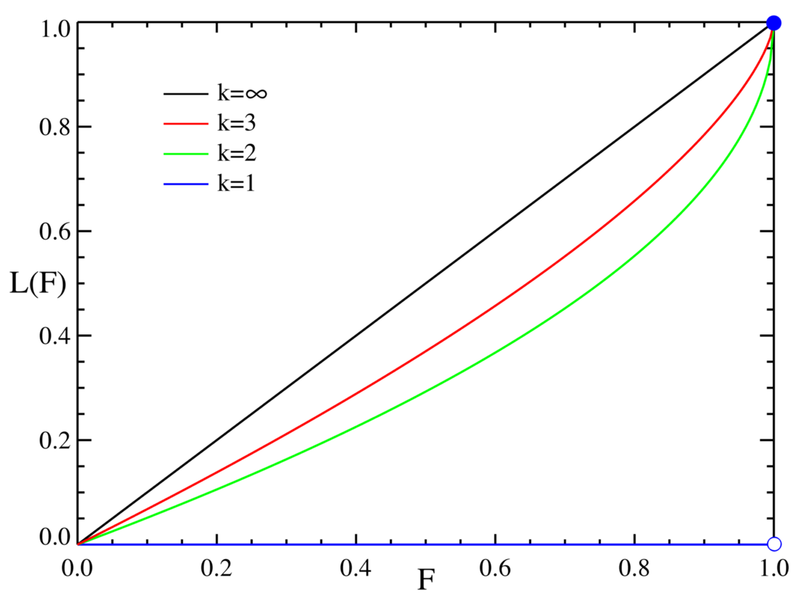Lorenz Curves Pareto Distributions

Compare Mathematica figure.

# Gini Coefficient for Pareto Distributions

$\begin{array}{c}G=\frac{1}{2\alpha -1}\end{array}$

Note: $\alpha =\infty$ → Gini = 0, and $\alpha =1$ → Gini = 1.

# Maximum Likelihood Estimation

The likelihood function for the Pareto index and cutoff parameters given a sample sample $x=\left({x}_{1},{x}_{2},...,{x}_{n}\right)$, is

$\begin{array}{c}L\left(\alpha ,{x}_{m}\right)=\prod _{i=1}^{n}\alpha \frac{{x}_{m}^{\alpha }}{{x}_{i}^{\alpha +1}}={\alpha }^{n}{x}_{m}^{n\alpha }\prod _{i=1}^{n}\frac{1}{{x}_{i}^{\alpha +1}}\end{array}$

Therefore, the loglikelihood function is

$\begin{array}{c}\ell \left(\alpha ,{x}_{m}\right)=nln\alpha +n\alpha ln{x}_{m}-\left(\alpha +1\right)\sum _{i=1}^{n}ln{x}_{i}\end{array}$

Estimating xm: $\ell \left(\alpha ,{x}_{m}\right)$ is strictly increasing in ${x}_{m}$. Since $x\ge {x}_{m}$, pick

$\begin{array}{c}{\stackrel{^}{x}}_{m}={min}_{i}{x}_{i}\end{array}$

# Estimating the Pareto Index

To find the estimator for $\alpha$, take the corresponding partial derivative and find $\alpha$ that makes it zero:

$\begin{array}{c}\frac{\partial \ell }{\partial \alpha }=\frac{n}{\alpha }+nln{x}_{m}-\sum _{i=1}^{n}ln{x}_{i}=0.\end{array}$

Thus the maximum likelihood estimator for $\alpha$ is:

$\begin{array}{c}\stackrel{^}{\alpha }=\frac{n}{\sum _{i}\left(ln{x}_{i}-ln{\stackrel{^}{x}}_{m}\right)}.\end{array}$

# Sampling from Power Law (Pareto) Distributions

• use inverse transform sampling:

draw from a uniform distribution $U$ (on (0,1]) and produce the Pareto variate $X$ as

$\begin{array}{c}X=\frac{{x}_{m}}{{U}^{1/\alpha }}\end{array}$

# Sampling from Power Law (Pareto) Distributions

```
nsamples = 10**6
u = 1 - np.random.random(nsamples)  # U(0,1]
x = u**(-1.0/(2.5 - 1.0))  # pareto distributed

```

# Sampling from Power Law (Pareto) Distributions

```
lastbin01 = 10
bins01 = np.linspace(1, lastbin01, 91) # bin size = 0.1
ind01 = np.digitize(x, bins01)
freq01 = np.bincount(ind01)[1:-1]    #trim the unbinned
relfreq01 = freq01 / float(nsamples)

```

# Sampling from Power Law (Pareto) Distributions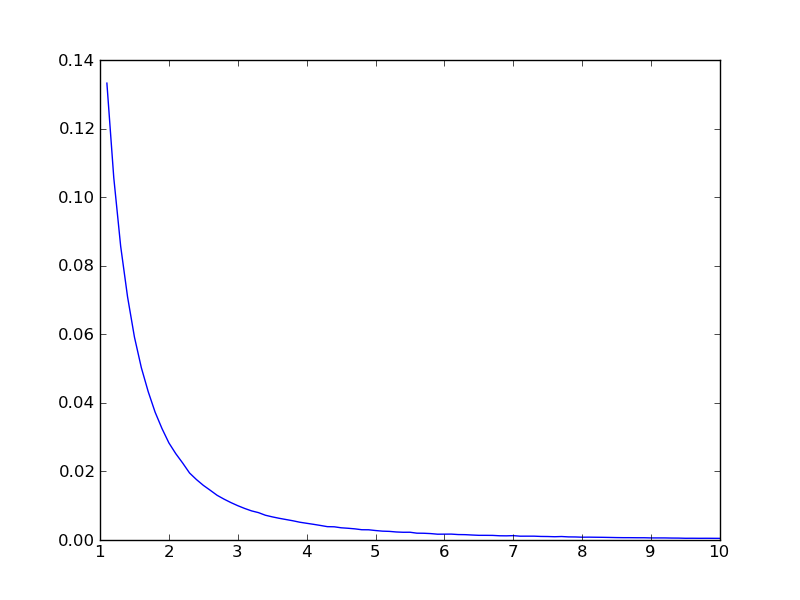Pareto Distribution: Samller Values are Common

# Sampling from Power Law (Pareto) Distributions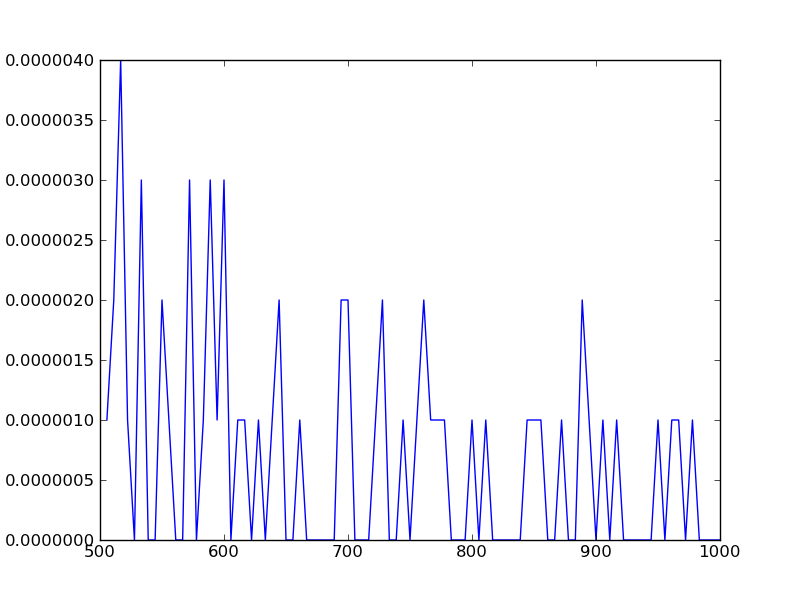Pareto Distribution: Larger Values are Rare